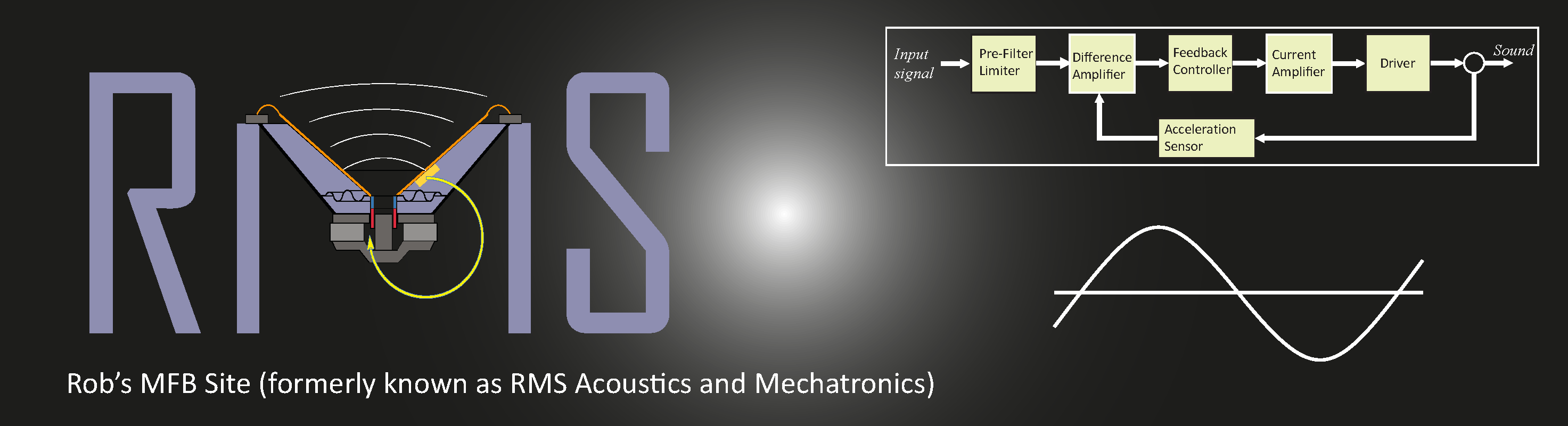Home Mechatronics About RMS DMC Sub Audio Design Education Kits & Parts

# The Design of High Performance Mechatronics (2nd edition)

The list is divided in three categories: (1) Critical errors and necessary additions that could lead to confusion and wrong conclusions, (2) Minor errors, like typo's that can be interpreted wrongly, calculation errors without significant impact on the conclusions or where the result is OK but not the method and (3) insignificant errors that do not interfere with the content, relate to a side remark, typos or a not 100% correct use of GB-English wording.

### 1: Critical errors and necessary additions

Page 57, 82: Due to professional blindness the magnetic permeability μ0 has been introduced and used many times thereafter, however its value has only once been mentioned in a formula. It should have been stated clearly at the introduction as μ0=4π·10-7 , while this is one of the very few exact numbers in physics. Also on page 82 with the introduction of electromagnetic waves, the propagation speed of electromagnetic waves should have been shown as c0=1/√0ε0), thereby showing the clear relationship between electricity and magnetism.

Page 76, Equation 2.49, second equation: k should be in the denominator and ω in de numerator, hence: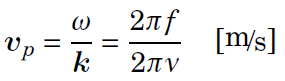as can be verified by dimension analysis.

Page 205, Sec 3.3.7.1: The definition of rigid-body mode by the uniform undeformed motion of this example of a suspension mode leads to confusion with students who learned that rigid-body motion only happens at 0 Hz, which is also discussed in Section 3.3.7. For this reason it is better to define this special situation as a "Quasi rigid-body suspension mode". The section is rewitten as follows: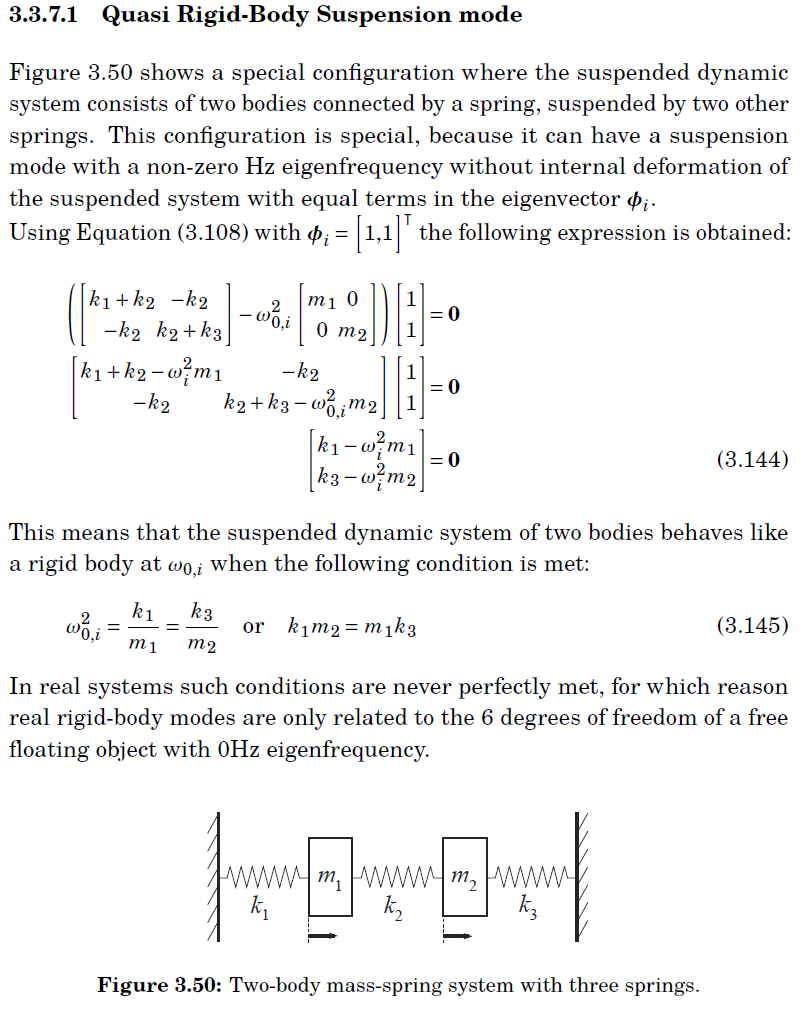Page 209, Figure 3.54: The dotted phase line of the rigid-body mode is placed at -360o while it should have been on -180o.

This error gives a fully different conclusion than what is presented. Even though the error is obvious it will lead to confusion with students who are not sufficiently acquainted with the minimum phase relation between a -2 slope and -180o phase.

Page 235, Start of the second paragraph: the sentence "...poles cannot create instability..." should of course have been "...zeros cannot create instability..."

Page 251 and further: The use of the term "open-loop" can cause confusion as it has two slightly different meanings.
As used in the first sections of Chapter 4, the term "open-loop" refers to the response of the output of a system to a signal at the input without closing a feedback path. In a fully described system like shown in Figure 4.5 it includes the input filter and feedforward path. In the stability analysis of negative feedback control, however, the term open-loop is used for the transfer function of the feedback loop itself from the error at the input of the feedback controller to the measured value. This second meaning of ``open-loop'' is only equal to the first open-loop definition, when both the input filter and measurement path are equal to one and the feedforward path is zero.

One could avoid the confusion by naming the second option differently like "loop-transfer function (LTF)" or "loop-gain" in the frequency domain, however as the term open-loop is often used in practice it is better to be aware of this difference in the same terminology.

### 2: Minor errors

Page 102: Fig. 2.31: red dashed line should be on the right edge of the yellow rectangle

Page 140: Eq (3.43): the ! should be ω

Page 145-146: Equation 3.60, first lines below Figure 3.16 and Equation 3.61 :

p1p2 should have been written in the denominator of Eq 3.6, Num' would than be Num/p1p2, further the reasoning remains valid, while Eq3.61 should have been: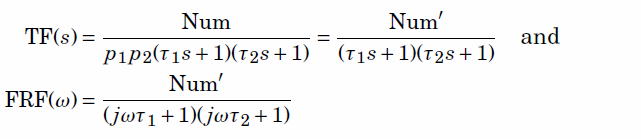Page 152:8: [. . . ] which determines the frequency stability of the resonator
at high Q levels.

Page 157: Eq (3.80) should be: m2s2x2(s) − k(x1 − x2)= 0

Page 209, 4th sentence below Figure 3.54: The reference should be Equation 3.121.

Page 232 and further: Negative stiffness poles (This error was also present in 1st edition....)

The description of poles with instable plants from page 232 and the magnetic bearing from page 280 suffered from an inconsistent use of the minus sign, although the numeric values of the outcome were correct.

With equation 4.1 the poles are determined as usual by calculating the values of s, where the denominator equals zero. With a positive stiffness these poles are imaginary as s equals plus or minus the square root of -k/m (minus!) to give a zero value for the denominator. The minus sign is missing in the term noted 3 lines below Equation 4.1. With a negative stiffness value the minus of the stiffness value will cancel the minus under the square root, resulting in real values for the poles.

In page 280 Section 4.3.6.1 this error was repeated, while mixing up the generic stiffness k with kn, which was meant to be the magnitude (104) of the negative stiffness k=-kn=-104. This should have been consistently applied in the simple calculations on page 281 and under Figure 4.28.

The terminology "Negative Stiffness" in Figure 4.28 can be better replaced by the pole values (√ kn/m ) and the value for the proportional control gain at that point (kp=0).

Page 217 Figure 3.60: The scaling of the first Nyquist plot should range from -10000 until + 10000 for both axes, iso -2000 until +2000 as was the case for the previous 3 figures.

Page 243 Equation 4.8: ω1 should be ω0

Page 250: paragraph before 4.3.1.1: [. . . ] unity-gain cross-over frequency fc = 1, 6Hz [. . . ]

Page 254, Equation 4.21: 1 should be replaced by in the numerator of the second term between the brackets

Page 256 - 258: On several places a notation from the first edition for the unity-gain cross-over frequency was not changed to the new notation, which was introduced for distinguishing it from a natural frequency in a closed-loop or passive system. For that reason f0 should be read as fc and ω1 should be read as ωc. Furthermore this has caused the last term in the upper and lower equations in the magnitude plot to be erroneous as 3ωc should have been 3fc and 0.33ωc should have been 0.33fc

Page 257: First PID guideline: The use of kt for the total gain of the controller at the unity-gain cross-over frequency fc is confusing as it may be interpreted with the taming of the differentiator. For that reason it can better be named kc, further the expression between brackets at the end of the first PID guideline contained an error by a slash (/) between 0.33 and kt. After replacing kt by kc and correcting the error, the first PID guideline reads as follows: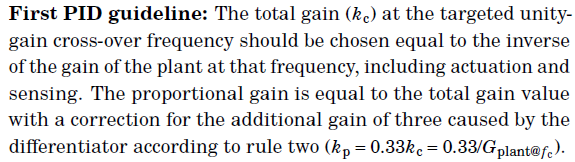Page 263, Equation 4.31: GS(s)=y/n=... should have been GS(s)=y/d=...

Page 263, Equations 4.32, 4.33: Some sign was wrong and the text should focus on control gain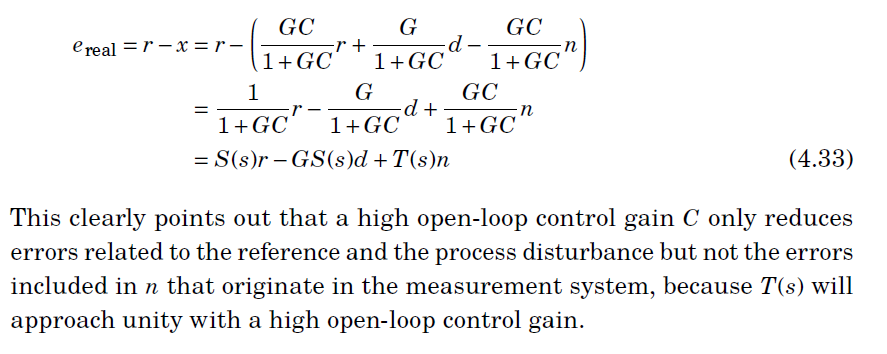Page 265, lines 1-2: complementary sensitivity S(s), should be T(s)

Page 267, gain margin, 5th sentence: The gain margin can have values between one and infinite. (below one the system is unstable)

Page 287, second paragraph 4th line: "of the previous section", should be "of Section 4.3.4".

Page 287, 4 lines above Figure 4.32: Figure 4.33 shows both the Bode plot and the Nyquist plot of this dynamic system, when combined with the PID controller that was determined in Section 4.3.4. The underlined text is missing.

Page 291: third line from the bottom of the page: the additional low-pass filter is the -3 slope of the complementary sensitivity function T(s) [. . . ]

Page 352, figure 5.11: The magneto motive force in the magnetic equivalent circuit fails μ in the denominator.

Page 355: Figure 5.13: At the left cross section of the horizontal H-axis with the curve "-H" should be "-Hc".

Page 360: First line should be : "also called the force or torque constant K, of the actuator.

Page 363, upper paragraph: A closing sentence is missing. It should be: "Also the return path of the magnetic field is much shorter and no longer requires the connecting structure of the two opposing magnet sections to be ferromagnetic."

Page 380:3: [. . . ] C-core version with two legs and the C E-core version
with three legs.

Page 384, Equation 5.80: A factor 2 is missing after replacing nI by Equation 5.79. The final result is still correct.

Page 397, Equation 5.101: The unit of resistivity should be Ω.m instead of Ω/m

Page 403, par 5.52, 6th sentence from below: The reference should be to Bibliography 56 and 60.

Page 404, above Equation 5.115: The power loss should be Pl=I2R, the squaring was missing.

Page 408:5-7: The reluctance of the Lorentz actuator is in any case considerably smaller higher than with the Reluctance and hybrid actuator because of their small air-gap.

Page 420, Equation 5.136: The d matrix should be d' (6 x 3), the transpose of d (3 x 6).
Related to this on page 421, while dij=d'ji Equation 5.139 is correct.

Page 435 Fig 5.54: The "0" on the magnitude scale should be 100

Page 457, Equation 6.25: The value of μr=100 is forgotten in the formula, thus the answer should have been L=125∙10-3 H.

Page 615: 7.4.2.2, second paragraph: [. . . ] combination of a spher ical and a cylindrical profile is an example of a shape that causes spherical aberration astigmatism.

Page 763 Figure 8.51: The caption mentions a green and blue curve while it has become black and blue.

Page 761/762: Eq. (8.51), (8.55), (8.56): mixed time-domain and s-domain, might cause confusion. Should be split into separate equations.

Page 773 Figure 8.58: The caption mentions a green and blue curve while it has become black and blue in the amplitude curve. The phase is OK.

Page 797, 3rd line above Equation 8.69: dy=dxsin2ϑ (the dx is missing), further in Equation 8.69 the factor two before ϑ has disappeared. while the equation should have started with dy=λ=dxsin2ϑ, where dy equals the one wavelength shift in the y-direction and dx the fringe distance.

3: Insignificant errors

Page 57: Figure 2.6: The vectors n and l in the drawing should have been noted in the new bold-italic notation (n, l)

Page 63: With James Watt the years of birth and death are forgotten (1736 - 1819)

Page 98 5th line from above: "unknown and with signals" should be "unknown signals"

Page 148: second paragraph: [. . . ] stored energy Es is equal to the maximimu maximum (peak)

Page 175, Equation 3.106 and 3.107: The zeros after the = should have been bold (0) as these are zero vectors. The same holds for Equation 3.131 on page 188 and the first 4 equations of Section 3.3.7 on page 202.

Page 205: The bold zeros in the equation of Section 3.3.7.1 are correct, but the equation number is missing.

Page 207: Figure 3.52: "with permission by" should be "with permission from".

Page 210: Figure 3.55: The horizontal dotted line @ 180 degrees on the phase plots is shifted down a little for some unkonown reason.

Page 235: "proper systems" should have been named "strictly proper systems" as having more poles than zeros.

Page 251: Preliminary PID guideline should be Preliminary first PID guideline and directly related to this error, page 257, just above First PID guideline: "zeroth guideline" should be "Preliminary first PID guideline".

Page 251 above Equation (4.16). The symbol for the control error (e) after "...until the measured sensor signal" should be placed after"...system from the input of the controller"

Page 285:16: the -1 point would be passed [. . . ]

Page 286: Figure 4.31: The horizontal dotted line @ 180 degrees on the phase plots is shifted down a little for some unknown reason.

Page 295, 8th line f.b.: "definitionanti-aliasing" should have been "anti-aliasing" referenced in the index. (missing Latex slash (\))

Page 314, last line before Multi-DOF Repr.....: "optimal pole location, as will be shown in..." should have been: as will be shown with the introduction of state feedback in Section 4.5.2.

Page 369: Fig. 5.23: ”/mathrm” shows up twice in the description (LaTeX error slash instead of backslash, which should place the typeface upright)

Page 418:17: [. . . ] at that location. This is illustrated in Figure 5.46.a.

Page 424, 6th sentence from below: The Dutch word "en" between magnitude and frequency should of course be "and"

Page 425, 3rd line from the bottom: the word "electric" should be left out.

Page 426: 5.7.4.1 "Piezoelectric"(capital)

Page 427, second sentence below Equation 5.142: voltage v should be capital V

Page 453, Equation 6.19. The brackets around (t) should be omitted sin(ωt) etc. and A1=Vp while A2=Ip.

Page 461, Figure 6.17: The numbering of the impedances has gone wrong with the resistors. Z2 should be Z3 and Z3 should be Z5

Page 508, Figure 6.49: The expression for the current sensing voltage has a wrong resitance in it. It should be Rcs instead of R3. Further the signs (+ and -) of the voltages are not consequently noted at the arrows. Plus is on top.

Page 509, Figure 6.50: Here Rs should have been Rcs

Page 646: three lines before section 7.5.4: reasoning is valid al as long as the transparent [. . . ]

Page 652: footnote 7: The term (NA) and (CD) are not [. . . ]

Page 657:3: [. . . ] lens material that shows an a large temperature dependency [. . . ]

Page 658: last paragraph, third line: [. . . ] index leading to wavefront errors. Especially with [. . . ]

Page 778 Last sentence before 8.8.1 should end with a dot.

Page 758: Figure 8.48: Analiser ==> Analyser

Page 795, below Equation 8.68, N in "where N equals" should have been written in Italic (parameter)

Page 779: Figure-Text 8.64, 3rd. line: … on  a scale with an equal grating period connected to…

Page 796: 3rd Text line: …constant value and with a movement. The signal will…

Page 829: Section 9.1.1, 3rd line: …with a certain width positione stationary with respect…

Page 862: Wafer curvature correction on a die should have been written with capitals.

© 2018 RMS Acoustics & Mechatronics